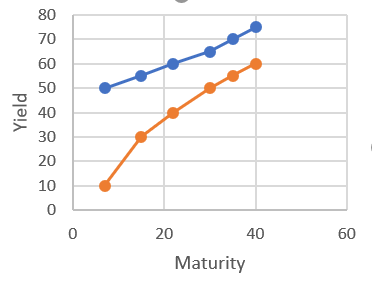# Assume that investors expect future short-run interest rates to fall. Show graphically and...

## Question:

Assume that investors expect future short-run interest rates to fall. Show graphically and explain how these expectations affect the yield curve.

## Yield Curve:

It shows yields or interest rate of bond with different maturity dates. The slope of a yield curve gives us a picture of future interest rate and economic balances.

Bond prices and interest rate have inverse relationship with each other. As bonds prices fall, interest rate rises and vice versa. When future short run interest rate falls, the yield curve would shift upward and start narrowing when maturity of years rises.